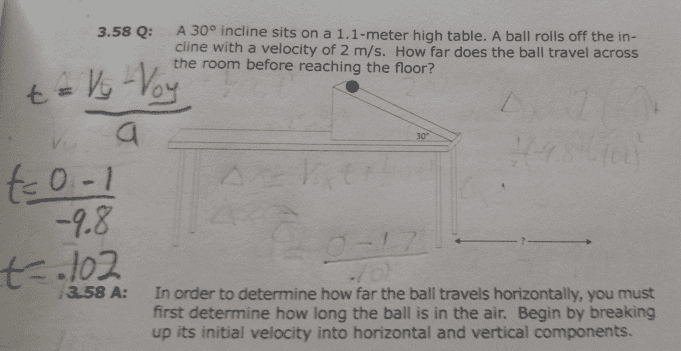# Kinematics problem: Ball rolling off ramp & table

• Bob Rudd
In summary, the conversation is about a problem involving a ball rolling off a table and the question of why the final velocity is not 0 when it reaches the floor. The discussion includes the use of equations and the misunderstanding of when the final velocity is 0.

## Homework Statement

http://imgur.com/0t8TVgq
http://imgur.com/0t8TVgq[Image copy inserted by moderator]
Sorry about all the erase marks. My question is why is the final velocity not 0.

V=Vo+at[/B]

## The Attempt at a Solution

So the question is asking for the horizontal distance the ball travels before it hits the floor. First to solve this I decided to solve for time. To do this is used V=Vo+ at which I rearranged to t=(V-Vo)/a. Using just the vertical component, I decided the vertical velocity of the ball when it reaches the floor is 0. So I did (0-1)/-9.8 which gave me a time of .102. The book solved for the final distance first and then solved for time
My question is why is the final velocity no just 0. When it reaches the floor it can't have a positive velocity because it has stopped moving.

Bob Rudd said:

## Homework Statement

http://imgur.com/0t8TVgq
http://imgur.com/0t8TVgq
Sorry about all the erase marks. My question is why is the final velocity not 0.

V=Vo+at[/B]

## The Attempt at a Solution

So the question is asking for the horizontal distance the ball travels before it hits the floor. First to solve this I decided to solve for time. To do this is used V=Vo+ at which I rearranged to t=(V-Vo)/a. Using just the vertical component, I decided the vertical velocity of the ball when it reaches the floor is 0. So I did (0-1)/-9.8 which gave me a time of .102. The book solved for the final distance first and then solved for time
My question is why is the final velocity no just 0. When it reaches the floor it can't have a positive velocity because it has stopped moving. [/B]

You know the ball falls for a certain distance when it rolls off the table. There's a SUVAT equation which deals with distance traveled under uniform acceleration, given an initial velocity. Why didn't you use this equation to find the time of the fall?

Bob Rudd said:
the vertical velocity of the ball when it reaches the floor is 0
No, that is after it has hit the floor. The equation you used is only valid for constant acceleration, so only until it is just about to touch the floor.